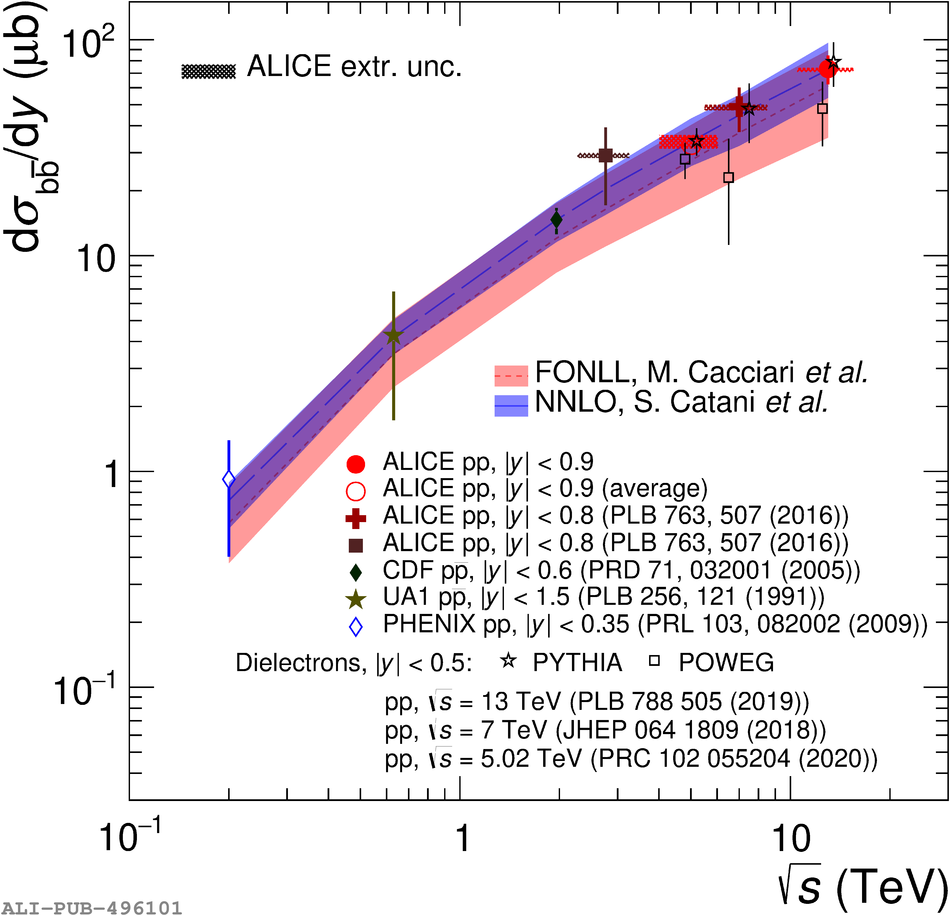# Figure 6

 The ${\rm d } \sigma_{\rm b \overline{\rm b}}/{\rm d}y$ at midrapidity as a function of the centre-of-mass energy. The ALICE measurement at \s = 5.02 TeV corresponds to the weighted average ofnon-prompt D mesons  and non-prompt \pJPsi (see text for details). The ALICE results are compared with existing measurements in pp collisions (PHENIX  and ALICE ) and in p$\overline{\rm p}$ collisions (UA1  and CDF ). The shaded area around the ALICE data points represents the extrapolation uncertainty. Results from dielectron measurements from the ALICE collaboration, obtained using either PYTHIA or POWHEG simulations, are also shown  FONLL  and NNLO  calculations in the rapidity range \$|y|